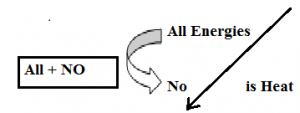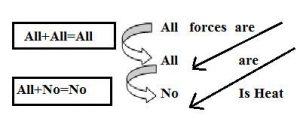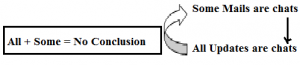# How to Solve Syllogism using Formula Method

Dear Banking Aspirant,

Syllogism is a topic which needs constant practice and different methods to solve the problems asked in the exams. Even though only 5 questions were asked from this topic , it is regular in all the exams and the exams without this topic is very less. This is a topic of utmost importance and cannot be left as such. Even though there have been many methods are used to solve the questions , this is one of the scoring part of all the candidates and they rush to this part in the exams to answer it first.

So learning this topic with the shortcuts and different methods helps a candidate to score well in this section which could eventually boost the overall score of the candidate other than the individual cutoff marks. So from this part , we are going to learn how to solve syllogism using formula method as it is the most widely used method after the Venn Diagram Method.

Why we should learn Syllogism using the Formula Method ? when everyone sticks with the conventional Venn Diagram method. Learning new concepts will actually adds strength to our devised strategy. Also if we can solve questions easier and faster with this method , there is nothing wrong in choosing this method ( Solving the Questions in the Exam is the key to get marks by utilizing less time ). So let’s brainstorm and learn a new method to solve syllogism.

Following are the main rules for solving Syllogism Problems

1. All+All=All
2. All+No=No
3. All+Some=No onclusion
4. Some+All=Some
5. Some+No= Some Not
6. Some+Some= No Conclusion
7. No +All = Some Not(Reversed)
8. No+Some=Some Not (Reversed)
9. No+No=No Conclusion
10. Some Not /Some Not Reversed +Anything = No Conclusion

If u want to convert any conclusion in Possibility

1. 11. If All A are B, then we can say – Some B are not A is a Possibility.
2. If Some B are not A, then we can say – All A are B is a possibility.
3. If Some A are B, then we can say – All A are B is a Possibility and All B are A is a Possibility.
4. All Some <———> Not Reversed
5. Some <———-> All
6. NO Conclusion = Any Possibility is true

Implications (In case of Conclusion from Single Statement)

 All <———> Some that means if All A are B then Some B are A is true Some  <———->   Some that means if Some A are B then Some B are A is true No  <———>  No that means if No A is B then NO B is A is true

How to solve questions using above rules?

There are two rules to solve syllogism questions –

Cross Cancellation and Vertical Cancellation

Example 1. Cross Cancellation

Statements

1.All forces are Energies

2.All Energies arePowers

3.No Power is Heat

Conclusions

1.No Energy is Heat

2.Some Forces being heat is Possibility

Solution –

Lets take 1st conclusion, we have to make relation between Energy and Heat so we will take statement 2 and 3This will be called Cross Cancelation, We have cancelled power from Power so we have left with (ALL+NO) Energies is/are Heat, and that is No Energy is Heat.

So Conclusion First is TRUE.

In second statement we have Force and Heat so we will need to make relation between force and heat. For this we need to take all 3 statementsNow we have left with ((All+All)+NO) Forces is/are Heat and that is NO Force is Heat . We don’t have any rule to convert this statement is Possibility so second conclusion is FALSE.

Example 2 – Vertical Cancellation

Statements:

1. Some Mails are Chats

Conclusion:

1. All Mails being update is a possibility

2. No update is Mail

Let’s take 1st Conclusion “All Mails Being Update is a Possibility” that means we have to make relation between Mails and UpdatesThis is called vertical cancellation. In this case direction of adding first phrase will be reversed i.e In Above example the conclusion will be (All+Some) Updates is/are Mails = No Conclusion .

If we get No Conclusion in case of Possibility then according to Rule No 16 possibility case will be definitely true . So Conclusion 1 follows and Conclusion 2nd Doesn’t follows.

SOME NOT REVERSED:

Let’s Take an Example

No A is B

All B is C

So the conclusion will be (No + All) A is C = (Some Not Reversed) A is C = Some C are Not A.